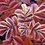# phi(phi(floor(exp(3!))))=96

This note's credits goes to a comment by Deepak Gowda in this post.

Generate 0 to 100 with only one digit once: the digit 3. You may use any other functions, like the one in the title.

Please enter as much as you can! :)Note by Kenny Lau
7 years ago

This discussion board is a place to discuss our Daily Challenges and the math and science related to those challenges. Explanations are more than just a solution — they should explain the steps and thinking strategies that you used to obtain the solution. Comments should further the discussion of math and science.

When posting on Brilliant:

• Use the emojis to react to an explanation, whether you're congratulating a job well done , or just really confused .
• Ask specific questions about the challenge or the steps in somebody's explanation. Well-posed questions can add a lot to the discussion, but posting "I don't understand!" doesn't help anyone.
• Try to contribute something new to the discussion, whether it is an extension, generalization or other idea related to the challenge.

MarkdownAppears as
*italics* or _italics_ italics
**bold** or __bold__ bold
- bulleted- list
• bulleted
• list
1. numbered2. list
1. numbered
2. list
Note: you must add a full line of space before and after lists for them to show up correctly
paragraph 1paragraph 2

paragraph 1

paragraph 2

[example link](https://brilliant.org)example link
> This is a quote
This is a quote
    # I indented these lines
# 4 spaces, and now they show
# up as a code block.

print "hello world"
# I indented these lines
# 4 spaces, and now they show
# up as a code block.

print "hello world"
MathAppears as
Remember to wrap math in $$ ... $$ or $ ... $ to ensure proper formatting.
2 \times 3 $2 \times 3$
2^{34} $2^{34}$
a_{i-1} $a_{i-1}$
\frac{2}{3} $\frac{2}{3}$
\sqrt{2} $\sqrt{2}$
\sum_{i=1}^3 $\sum_{i=1}^3$
\sin \theta $\sin \theta$
\boxed{123} $\boxed{123}$

Sort by:

$\lfloor \sin(3^{\circ})\rfloor = 0$

$\text{sgn}(3) = 1$ (sign function)

$\phi(3) = 2$ (Euler's totient phi function)

$3$

$\sigma(3) = 4$ (divisor sum)

$\Sigma(3) = 5$ (prime sum)

$3! = 6$

$\lfloor \csc(3)\rfloor = 7$

$\lceil \csc(3)\rceil = 8$

$\lfloor\text{antilog}(\tan(3)) \rfloor = 9$ ($\text{antilog}(x) = 10^{x}$)

$\Sigma(\Sigma(3)) = 10$

$p_{\Sigma(3)} = 11$ (n-th prime number)

$\sigma(3!) = 12$

$F_{\lceil \csc(3)\rceil} = 13$ (Fibonacci function $F_{1} = 0, F_{2} = 1, F_{n+2} = F_{n} + F_{n+1}$)

$\sigma(F_{\lceil \csc(3)\rceil}) = 14$

$\sigma(\lceil \csc(3)\rceil) = 15$

$\phi(s(\sigma(\sigma(\sigma(3))))) = 16$

$s(\sigma(\sigma(\sigma(3)))) = 17$ (aliquot sum $s(n) = \sigma(n) - n$)

$\sigma(s(\sigma(\sigma(\sigma(3))))) = 18$

$p_{\lceil \csc(3)\rceil} = 19$

$\sigma(p_{\lceil \csc(3)\rceil}) = 20$

$\lfloor \tan(\tan(\cos(\sin(3^{\circ})))) \rfloor = 21$

$\lceil \tan(\tan(\cos(\sin(3^{\circ})))) \rceil = 22$

$p_{\lfloor\text{antilog}(\tan(3)) \rfloor} = 23$

$(\Sigma(3))! = 24$

Actually we can do any prime numbers if we have first 25 numbers. And also prime numbers minus 1 using Euler's totient function. And also Fibonacci numbers. And also Lucas numbers. And blahuhuhuh

• $\left\lfloor{cotan(3°)}\right\rfloor=20$
• $\left\lceil{cotan(3°)}\right\rceil=19$
• $\mu(\mu(3))=1$
• $\frac{d}{dx}(3)=0$
• σ(3)=4 where σ(n) is sum of positive divisors.

- 7 years ago

Well, $\frac d{dx}(3)=0$.

- 7 years ago

oops yeah...its 0 mis-typo...

- 7 years ago

The floor and ceiling is also switched too.

I've commented but you've created...

so credits goes to Kenny Lau and Vinay Sipani

- 7 years ago

Ty... But the credit must go to you..

- 7 years ago